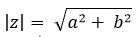Engineering functions arithmetic operations of complex numbers

Excel Function COMPLEX

The COMPLEX() function takes real and imaginary coefficients and converts them to a complex number.

The complex number so formed is of the form X+iY.

The third parameter of the function describes the suffix to be used; either "I" or "j".

The default suffix is "I".

 Real Part Imaginary Part COMPLEX() default suffix COMPLEX() with "j" Formula 2 5 2+5i 2+5j =COMPLEX(L4,M4,"j") 4 2.3 4+2.3i 4+2.3j =COMPLEX(L5,M5,"j") 6 5.7 6+5.7i 6+5.7j =COMPLEX(L6,M6,"j") 1.4 8 1.4+8i 1.4+8j =COMPLEX(L7,M7,"j") 1.7 11 1.7+11i 1.7+11j =COMPLEX(L8,M8,"j")

Excel Function IMABS

The IMABS() function is used to determine the absolute value of a complex number.

The absolute value is also called as modulus.

It is nothing but the square root of sum of square of imaginary part and square of real part.

For example, for a complex number a+ib, the absolute value is determined as below:Complex number IMABS Formula 2+5i 5.3851648071345 =IMABS(L18) 4+2.3i 4.61410879802373 =IMABS(L19) 6+5.7i 8.27586853448023 =IMABS(L20) 1.4+8i 8.12157620169878 =IMABS(L21) 1.7+11i 11.1305884839931 =IMABS(L22)

Excel Function IMAGINARY

The IMAGINARY() function is used to determine the imaginary coefficient of the complex number.

This function does not return the suffix "I" or "j" and only returns the number.

The complex numbers are always treated as text and hence should be specified as a string within the function.

 Complex number IMAGINARY Formula 2+5i 5 =IMAGINARY(L33) 4-2.3i -2.3 =IMAGINARY(L34) 6+5.7i 5.7 =IMAGINARY(L35) 1.4-8i -8 =IMAGINARY(L36) 1.7+11i 11 =IMAGINARY(L37)

Excel Function IMARGUMENT

A complex number can also be expressed in terms of angle in radians.

The IMARGUMENT() function is useful in such scenario where the angle in radians is to be determined.

This function takes the complex number in normal form as its parameter.

 Complex number IMARGUMENT Formula 2+5i 1.19028994968253 =IMARGUMENT(L46) 4-2.3i -0.52183427981441 =IMARGUMENT(L47) 6+5.7i 0.759762754875771 =IMARGUMENT(L48) 1.4-8i -1.39755066034253 =IMARGUMENT(L49) 1.7+11i 1.41746393839657 =IMARGUMENT(L50)

Excel Function IMCONJUGATE

The IMCONJUGATE() function is used to determine the complex conjugate of the given complex number.

The complex conjugate is determined by inversing the sign of the imaginary number.

This function is most often used in signal processing problems.

 Complex number IMCONJUGATE Formula 2+5i 2-5i =IMCONJUGATE(L59) 4-2.3i 4+2.3i =IMCONJUGATE(L60) 6+5.7i 6-5.7i =IMCONJUGATE(L61) 1.4-8i 1.4+8i =IMCONJUGATE(L62) 1.7+11i 1.7-11i =IMCONJUGATE(L63)

Excel Function IMREAL

The IMREAL() function is used to determine the real part of the complex number.

The real part, if negative, is returned as a negative number.

This function takes a single complex number as its argument.

 Complex number IMREAL Formula 2+5i 2 =IMREAL(L72) 4-2.3i 4 =IMREAL(L73) 6+5.7i 6 =IMREAL(L74) 1.4-8i 1.4 =IMREAL(L75) 1.7+11i 1.7 =IMREAL(L76)